﻿F Test

F Test for Variances Calculator

Two population variances comparison

Target: To check if the difference between the average (mean) of two groups (populations) is significant, using sample data

Example1: A man of average hight is expected to be 10cm taller than a woman of average hight (d=10)

Example2: The average weight of an apple grown in field 1 is expected to be equal in weight to the average apple grown in field 2 (d=0)

Assumptions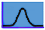The population's distribution is Normal

Required Sample Data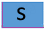S1,SS2 -Sample standard deviation of the population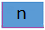n1,n2 - Sample size of group1 and group2

Enter input data and then press "Calculate test" button to get the results

Hypotheses

 H0: σ12 ≥ σ22 H0: σ12 = σ22 H0: σ12 ≤ σ22 H1: σ12 < σ22 H1: σ12 ≠ σ22 H1: σ12 > σ22

Test statisticF distribution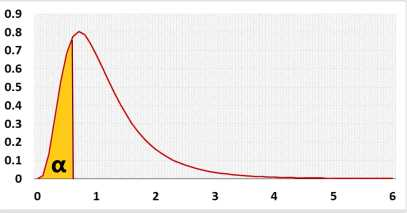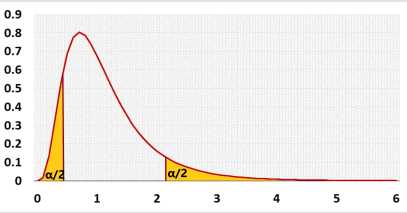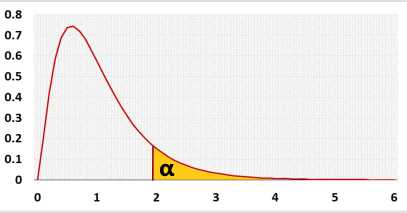Test calculation

If you enter raw data, the tool will run the Shapiro-Wilk normality test and calculate outliers, as part of the test calculation.

Statistic Data

 α: Significant level (0-1), maximum chance allowed rejecting H0 while H0 is correct (Type1 Error) Outliers: IncludedExcluded It is not recommended to exclude outliers unless you know the reasons
or or enter summarized data ( n, S) in Group1 and Group2 forms

Enter sample data

Header: You may change groups' name to the real names.
Data: When entering data, press Enter after each value

It is okay to leave empty cells, empty cells or non numeric cells won't be counted

Enter sample data

You may copy data from Excel, Google sheets or any tool that separate data with Tab and Line Feed.
Copy the data, one block of 3 consecutive columns includes the header, and paste below.

It is okay to leave empty cells, empty cells or non numeric cells won't be counted

Group l:

 n1: Sample size of group1 S1: Sample Standard deviation of group1 skewness: Normality pval1: Shapiro Wilk test Outliers count: ,based on the Tukey's fences method, k=1.5

Group 2:

 n2: Sample size of group2 S2: Sample Standard deviation of group2 skewness: Normality pval2: Shapiro Wilk test Outliers count: ,based on the Tukey's fences method, k=1.5

validation message